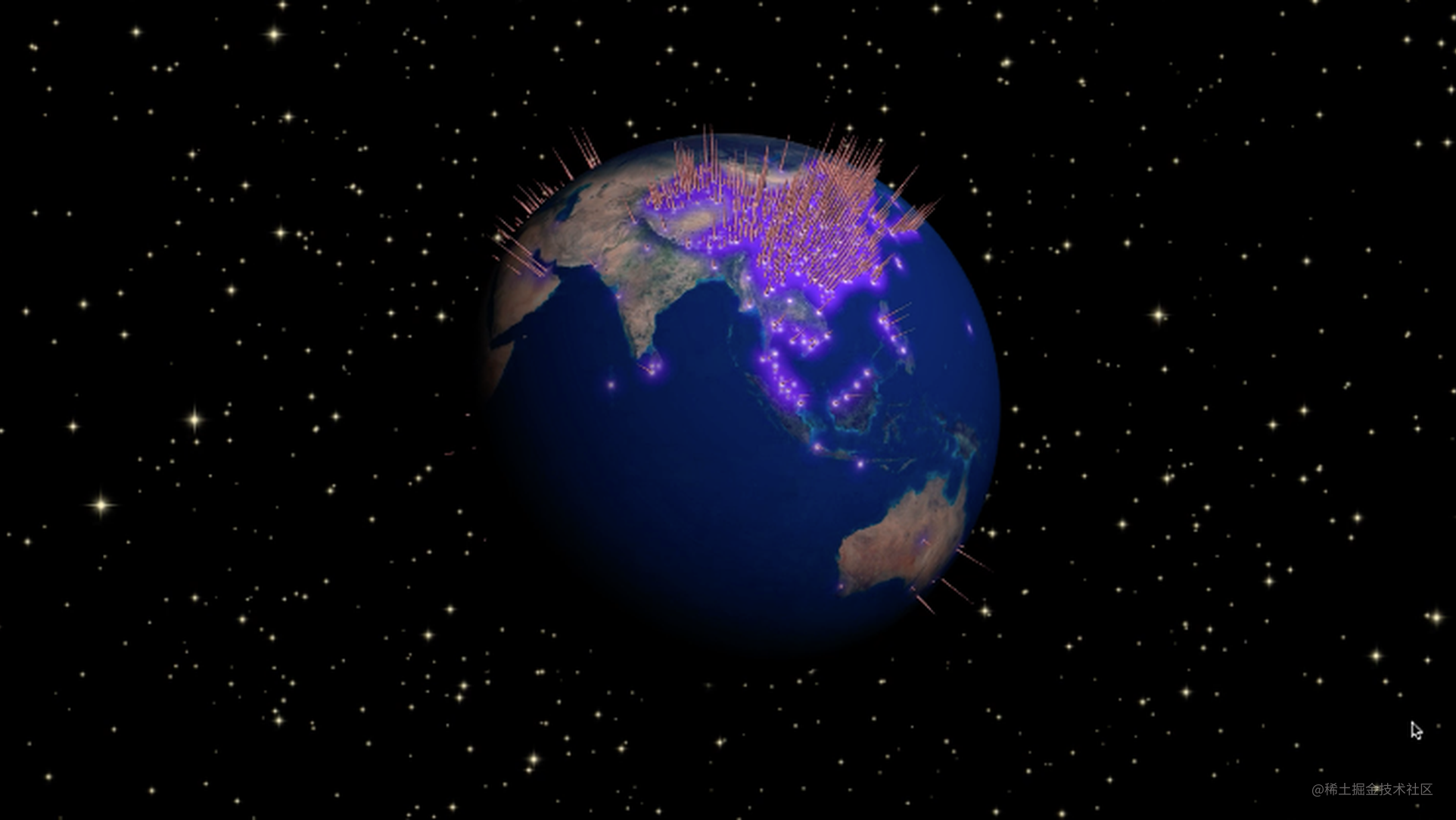# 用代码构建星辰大海数据体验技术团队（ProtoTeam） @ 蚂蚁金服（杭州）网络技术有限公司

—阿里人的宣言

embed: sky.mov

## 结构分解

### 星空

``````var starts = [];
for (let i = 0; i < 10000; i++) {
starts.push({
x: Math.random() * width,
y: Math.random() * height,
z: Math.random() * depth,
//当前进度 0 - 1, 当前大小按  (1 - t) * minSize + t * maxSize
t: Math.random(),
//变化方向
direction: Math.random(),
//变化步长 暂定为常量 后面可以根据需要每个星星都不一样
step: 0.01,
//最小
minSize: 10,
//最大
maxSize: 20
});
}
animate();
function animate () {
requestAnimationFrame(animate);
starts.forEach((item) => {
let { t, minSize, maxSize } = item;
//计算当前尺寸
item.size = (1 - t) * minSize + t * maxSize;
//改变进度
if (t > 1) {
//如果已经最大了 则开始减少
item.direction = -1;
} else if (t < 0) {
//最小了 则开始变大
item.direction = 1;
}
//修改进度
item.t += item.step * item.direction;
});
//根据上面的值进行渲染
render();
}

``````//流星处理逻辑
var falls = [];
for (let i = 0; i < 10; i++) {
falls.push({
//流星的起点
start: {
x: Math.random() * width,
y: Math.random() * height,
z: Math.random() * depth
},
//流星的终点
end: {
x: Math.random() * width,
y: Math.random() * height,
z: Math.random() * depth
},
//当前进度 0 - 1, 当前位置按 二次贝塞尔曲线计算即可
t: Math.random(),
step: 0.01
});
}

function animate () {
requestAnimationFrame(animate);
starts.forEach((item) => {
let { t, start as p0, end as p2} = item;
//随意设置一个控制点
let p1 = {
x: p0.x,
y: (p0.y + p2.y) / 2,
z: p2.z
};
//按二次贝塞尔曲线 计算当前位置
//(1 - t) * (1 - t) * p0 + 2 * t * (1 - t ) * p1  + t * t * p2

let tmp = {
x: (1 - t) * (1 - t) * p0.x + 2 * t * (1 - t ) * p1.x + t * t * p2.x,
y: (1 - t) * (1 - t) * p0.y + 2 * t * (1 - t ) * p1.y + t * t * p2.y,
z: (1 - t) * (1 - t) * p0.z + 2 * t * (1 - t ) * p1.z + t * t * p2.z
};
item.t += item.step;
item.tmp = tmp;
//改变进度
if (t > 1) {
//如果已经最大了变为0
item.t = 0;
//重置起始点
item.start = {
x: Math.random() * width,
y: Math.random() * height,
z: Math.random() * depth
};
item.end = {
x: Math.random() * width,
y: Math.random() * height,
z: Math.random() * depth
};
}
});
//根据上面的值进行渲染
render();
}

### 地球

``````  //经纬度转x,y平面坐标
lnglatToXY ({lng, lat}, width, height) {
let x = (lng - (-180)) / 360 * width;
let y = Math.abs((lat - 90) / 180) * height;
return {
x,y
};
}

``````    //渲染纹理
async function render () {
//全球背景图片
//热点背景图片
//画大背景
ctx.drawImage(worldBg, 0, 0, width, height);
//按经纬度绘制热点
data.forEach((item) => {
let lng = item.lnglat;
let lat = item.lnglat;
let {x, y} = util.lnglatToXY({lng, lat}, width, height);
ctx.drawImage(hotBg, x - size / 2, y - size / 2, size, size);
});
//生产纹理，然后 直接映射蒙到球上就可以了
let texture = new THREE.Texture(canvas);
texture.needsUpdate = true;
return texture;
}

ok，到这里就地球就生成了。后面就让地球每帧绕y轴旋转既可

``````animate();
function animate () {
requestAnimationFrame(animate);
earth.rotation.y += 0.01;
render();
}

### 交易线

ok 旋转的地球也有了， 下面就是要绘制 地球上的交易线了。还是首先要有经纬度 到空间坐标的一个转换。 具体原理见下面。

``````  //经纬度转空间坐标的具体代码, r 为球体半径
lnglatToXYZ ({lng, lat}, r) {
var phi = (90 - lat) * Math.PI / 180;
var theta = -1 * lng * Math.PI / 180;
return {
x: r * Math.sin(phi) * Math.cos(theta),
y: r * Math.cos(phi),
z: r * Math.sin(phi) * Math.sin(theta)
};
}

``````//原始数据
let lines = [
{lng, lat },
{lng, lat },
....
];

//生成一些控制参数
lines.forEach((item) => {
//控制点 0 - 1 用来计算直线的终点 t * length 这里随机数是为了 不同的线初始状态不同
item.t = Math.random();
//变化方向
item.direction = Math.random() > 0.5 ? 1 : -1;
//变化速度 暂定都一样
item.step = 0.01;
//线段的长度 这里可以根据实际数据 比如 value来映射长度 本出为了示意 用随机数了
item.length =  Math.random() * r;
});

animate();
function animate () {
requestAnimationFrame(animate);
lines.forEach((item) => {
let p1 = lnglatToXYZ(item.lng, item.lat, r);
//当前的终点 用 t
let p2 = lnglatToXYZ(item.lng, item.lat, r + item.length * t);
//根据 p1, p2 即可绘制一条直线
if (item.t > 1) {
item.direction = -1;
} else if (item.t < 0) {
item.direction = 1;
}
item.t += item.direction * item.step;
});
render();
}

ok 以上就是 闪烁的繁星， 天边划过的流星， 旋转的地球， 呼吸的交易线 按对应位置组合在一起， 就是星辰大海了。 当然 看到这里 你可能会问， 星辰有了 但是 说好的大海呢， 其实有的 你仔细看 地球表面都是水啊，那就是海。